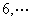2．多变量函数的极值

[极值(极大值或极小值)]  设函数

y= f (x1,x2,)= f (x)

0<|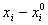|<δ,i=1,2,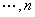f (x)< f (x0)    ( f (x)> f (x0))

[极值存在的必要条件]  假定函数f (x)在区域D内存在有限偏导数.若在点x0(D)处函数有极值，则必有

`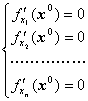（2）`

[极值存在的充分条件(二元函数的情形)]  设点x0=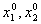）为函数y= f (x1,x2)的稳定点，并且函数f (x1,x2)在稳定点x0的邻域内有定义，连续，并有一阶及二阶连续偏导数.引进记号k = p1+p2

D1=,D2=D1>0    D2>0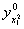>0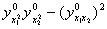>0

ii）稳定点x0是极大点的充分条件是：

D1<0    D2>0<0>0

D2<0，则x0不是极值点，当D2=0时不能肯定x0是否极值点，必须考察更高阶的偏导数.

[极值存在的充分条件(一般情形)]  设点x0=(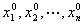)为函数y= f (x)= f (x1,x2,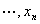)的稳定点，并且函数f (x)在稳定点x0的邻域内有定义，连续，并有一阶及二阶连续偏导数.引进记号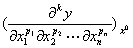k =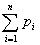Din个变量依次计算n个行列式D1,D2,…,Dn.那末

i）稳定点x0是极小点的充分条件是：所有的行列式都是正的，即

Di>0,  i=1,2,ii）稳定点x0是极大点的充分条件是：所有标号为偶数的行列式是正的，所有标号为奇数的行列式是负的，即

Di<0,  i=1,3,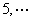Di>0,  i=2,4,